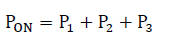# Calculation of Average Loss

## Calculation of Average Loss

This section describes the calculation of the average loss when the actual waveform is converted to an approximate waveform. Calculate the total average loss by summing the average losses for each block.
The following figure shows an example of an approximate waveform.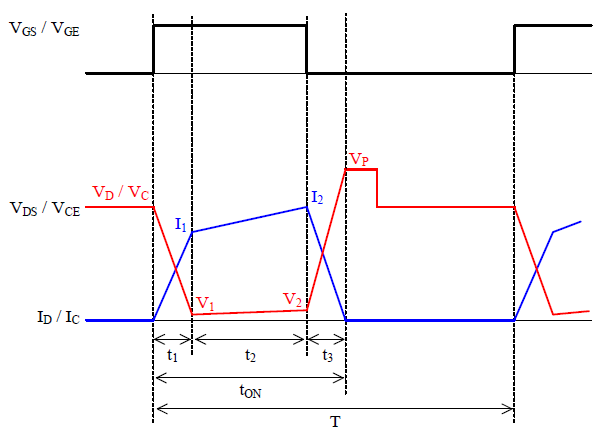Where:
t1 is switching loss time at turn-on,
t2 is switching loss time at saturation,
t3 is switching loss time at turn-off,
tON is on-time, and
T is period.

The average loss, P1, for the t1 period is calculated by the following equation.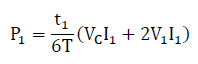The average loss, P2, for the t2 period is calculated by the following equation.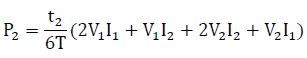The average loss, P3, for the t3 period is calculated by the following equation.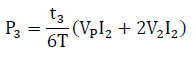Thus, the total average loss, PON, is calculated by the following equation.